Next: TWO EXAMPLES OF RAYMOND Up: APPLICATIONS OF CIRCUMSCRIPTION Previous: WHETHER BIRDS CAN FLY

# THE UNIQUE NAMES HYPOTHESIS

Raymond Reiter (1980b) introduced the phrase ``unique names hypothesis'' for the assumption that each object has a unique name, i.e. that distinct names denote distinct objects. We want to treat this nonmonotonically. Namely, we want a wff that picks out those models of our initial assumptions that maximize the inequality of the denotations of constant symbols. While we're at it, we might as well try for something stronger. We want to maximize the extent to which distinct terms designate distinct objects. When there is a unique model of the axioms that maximizes distinctness, we can put it more simply; two terms denote distinct objects unless the axioms force them to denote the same. If we are even more fortunate, as we are in the examples to be given, we can say that two terms denote distinct objects unless their equality is provable.

We don't know a completely satisfactory way of doing this. Suppose that we have a language L and a theory T consisting of the consequences of a formula A. It would be most pleasant if we could just circumscribe equality, but as Etherington, Mercer and Reiter (1985) point out, this doesn't work, and nothing similar works. We could hope to circumscribe some other formula of L, but this doesn't seem to work either. Failing that, we could hope for some other second order formula taken from L that would express the unique names hypothesis, but we don't presently see how to do it.

Our solution involves extending the language by introducing the names themselves as the only objects. All assertions about objects are expressed as assertions about the names.

We suppose our theory is such that the names themselves are all provably distinct. There are several ways of doing this. Let the names be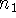,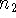, etc. The simplest solution is to have an axiom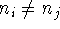for each pair of distinct names. This requires a number of axioms proportional to the square of the number of names, which is sometimes objectionable. The next solution involves introducing an arbitrary ordering on the names. We have special axioms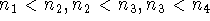, etc. and the general axioms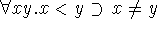and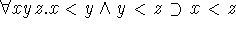. This makes the number of axioms proportional to the number of names. A third possibility involves mapping the names onto integers with axioms like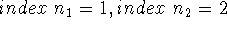, etc. and using a theory of the integers that provides for their distinctness. The fourth possibility involves using string constants for the names and ``attaching'' to equality in the language a subroutine that computes whether two strings are equal. If our names were quoted symbols as in LISP, this amounts to having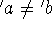and all its countable infinity of analogs as axioms. Each of these devices is useful in appropriate circumstances.

From the point of view of mathematical logic, there is no harm in having an infinity of such axioms. From the computational point of view of a theorem proving or problem solving program, we merely suppose that we rely on the computer to generate the assertion that two names are distinct whenever this is required, since a subroutine can easily tell whether two strings are the same.

Besides axiomatizing the distinctness of the constants, we also want to axiomatize the distinctness of terms. This may be accomplished by providing for each function two axioms. Letting foo be a function of two arguments we postulate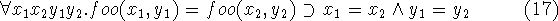and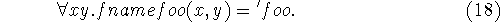The first axiom ensures that unless the arguments of foo are identical, its values are distinct. The second ensures that the values of foo are distinct from the values of any other function or any constant, assuming that we refrain from naming any constant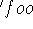.

These axioms amount to making our domain isomorphic to an extension of the Herbrand universe of the language.

Now that the names are guaranteed distinct, what about the objects they denote? We introduce a predicate e(x,y) and axiomatize it to be an equivalence relation. Its intended interpretation is that the names x and y denote the same object. We then formulate all our usual axioms in terms of names rather than in terms of objects. Thus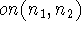means that the object named byis on the object named by, and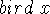means that the name x denotes a bird. We add axioms of substitutivity for e with regard to those predicates and functions that are translates of predicates referring to objects rather than predicates on the names themselves. Thus for a predicate on and a function foo we may have axioms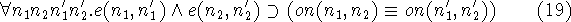and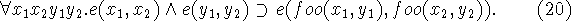If for some class C of names, we wish to assert the unique names hypothesis, we simply use an axiom like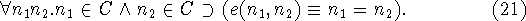However, we often want only to assume that distinct names denote distinct objects when this doesn't contradict our other assumptions. In general, our axioms won't permit making all names distinct simultaneously, and there will be several models with maximally distinct objects. The simplest example is obtained by circumscribing e(x,y) while adhering to the axiom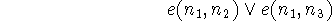where,, andare distinct names. There will then be two models, one satisfying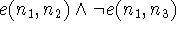and the other satisfying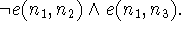Thus circumscribing e(x,y) maximizes uniqueness of names. If we only want unique names for some class C of names, then we circumscribe the formula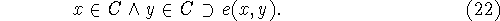An example of such a circumscription is given in Appendix B. However, there seems to be a price. Part of the price is admitting names as objects. Another part is admitting the predicate e(x,y) which is substitutive for predicates and functions of names that really are about the objects denoted by the names. e(x,y) is not to be taken as substitutive for predicates on names that aren't about the objects. Of these our only present example is equality. Thus we don't have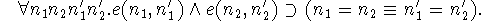The awkward part of the price is that we must refrain from any functions whose values are the objects themselves rather than names. They would spoil the circumscription by not allowing us to infer the distinctness of the objects denoted by distinct names. Actually, we can allow them provided we don't include the axioms involving them in the circumscription. Unfortunately, this spoils the other property of circumscription that lets us take any facts into account.

The examples of the use of circumscription in AI in the rest of the paper don't interpret the variables as merely ranging over names. Therefore, they are incompatible with getting unique names by circumscription as described in this section. Presumably it wouldn't be very difficult to revise those axioms for compatibility with the present approach to unique names.Next: TWO EXAMPLES OF RAYMOND Up: APPLICATIONS OF CIRCUMSCRIPTION Previous: WHETHER BIRDS CAN FLY

John McCarthy
Sat Jun 1 13:54:22 PDT 1996